# NCERT Solution for Class 10 Mathematics Chapter 6 Triangles

 Chapter Name NCERT Solution for Class 10 Maths Chapter 6 Triangles Topics Covered Short Revision for the ChapterNCERT Exercise Solution Related Study NCERT Solution for Class 10 MathsNCERT Revision Notes for Class 10 MathsImportant Questions for Class 10 MathsMCQ for Class 10 MathsNCERT Exemplar Questions For Class 10 Maths

## Short Revision for Triangles

1. Similar figures have the same shape.
2. Congruent figures have the same shape and same size.
3. Congruent figures are also the similar figures.
4. Similar figures are not necessarily the congruent figures.
5. All circles are similar.
6. All squares are similar.
7. All equilateral triangles are similar.
8. Two photographs of the same size and of the different persons are not similar.
9. If one figure is similar to another figure and this second figure is similar to a third figure, then the first figure is similar to the third figure.
10. Two triangles are similar, if their corresponding angles are equal and their corresponding sides are in the same ratio.
11. If s line is drawn parallel to one side of a triangle to intersect the other two sides in distinct points, then the other two sides are divided in the same ratio (Basic Proportionality Theorem).
12. If a line divides any two sides of a triangle in the same ratio, then the line is parallel to third side (converse of Basic Proportionality Theorem).
13. If the corresponding sides of two triangles are in the same ratio, then the triangles are similar (SSS similarity criterion).
14. If the corresponding angles of two triangles are equal, then the triangles are similar (AAA similarity criterion)
15. If the two angles of one triangle are equal to the two angles of another triangle, then the two triangles are similar (AA similarity criterion).
16. If one angle of a triangle is equal to one angle of another triangle and the sides including these angles are in the same ratio, then the triangles are similar (SAS similarity criterion).
17. The ratio of the areas of two similar triangles is equal to the ratio of squares of
(i) any two corresponding sides
(ii) their perimeters
(iii) any two corresponding altitudes
(iv) any two corresponding medians
(v) any two corresponding angles bisector segments.
18. If a perpendicular is drawn from the vertex of the right angle of a right triangle to the hypotenuse, then it divides the triangle into two mutually similar triangles and each of them is similar to the whole triangle.
19. The square of he hypotenuse of a right - triangle is equal to the sum of the square of the remaining two sides (Pythagoras Theorem).
20. In a triangle, if square of one side is equal to the sum of the squares of the other two sides, then the angle opposite the first side is a right angle.
21. Any median of a triangle divides it into two triangles of equal areas.

### NCERT Exercise Solutions

#### Exercise 6.1

1. Fill in the blanks using correct word given in the brackets:-
(i) All circles are __________. (congruent, similar)
(ii) All squares are __________. (similar, congruent)
(iii) All __________ triangles are similar. (isosceles, equilateral)
(iv) Two polygons of the same number of sides are similar, if
(a) their corresponding angles are __________ and
(b) their corresponding sides are __________. (equal, proportional)

Solution

(i) similar
(ii) similar
(iii) equilateral
(iv) equal, proportional.

2. Give two different examples of pair of
(i) Similar figures
(ii) Non-similar figures

Solution
(i) (a) A passport size photograph and a postcard size photograph of the same person from the same negative.
(b) Equilateral triangles.
(ii) (a) A square and a rhombus.
(b) A circular dinning table and a rectangular dinning table.

3. State whether the following quadrilaterals are similar or not:

Solution
Not similar as corresponding angles are not equal.

#### Exercise 6.2

1. In figure. (i) and (ii), DE || BC. Find EC in (i) and AD in (ii).

2. E and F are points on the sides PQ and PR respectively of a ΔPQR. For each of the following cases, state whether EF || QR.
(i) PE = 3.9 cm, EQ = 3 cm, PF = 3.6 cm and FR = 2.4 cm
(ii) PE = 4 cm, QE = 4.5 cm, PF = 8 cm and RF = 9 cm
(iii) PQ = 1.28 cm, PR = 2.56 cm, PE = 0.18 cm and PF = 0.63 cm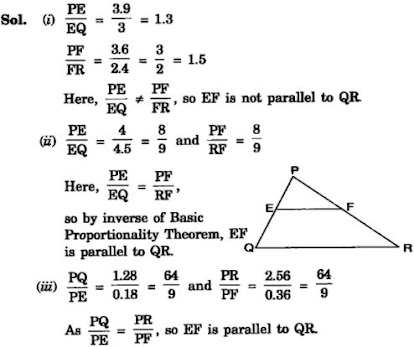3. In the figure, if LM || CB and LN || CD, prove that AM/AB = AN/AD

Solution
Given : In the given figure, LM॥ BC and LN॥ CD.
To prove : AM/AB = AN/AD.
Proof : In △ABC, LM॥BC

4. In the figure, DE||AC and DF||AE. Prove that BF/FE = BE/EC

5. In the figure, DE||OQ and DF||OR, show that EF||QR.

Solution
Proof : In △PQO, DE॥OQ.

6. In the figure, A, B and C are points on OP, OQ and OR respectively such that AB || PQ and AC || PR. Show that BC || QR.

7. Using Basic proportionality theorem, prove that a line drawn through the mid-points of one side of a triangle parallel to another side bisects the third side. (Recall that you have proved it in Class IX).

8. Using Converse of basic proportionality theorem, prove that the line joining the mid-points of any two sides of a triangle is parallel to the third side. (Recall that you have done it in Class IX).

9. ABCD is a trapezium in which AB || DC and its diagonals intersect each other at the point O. Show that AO/BO = CO/DO.

10. The diagonals of a quadrilateral ABCD intersect each other at the point O such that AO/BO = CO/DO. Show that ABCD is a trapezium.

#### Exercise 6.3

1. State which pairs of triangles in Figure, are similar. Write the similarity criterion used by you for answering the question and also write the pairs of similar triangles in the symbolic form:

2. In the figure, ΔODC ∝ ¼ ΔOBA, ∠ BOC = 125° and ∠ CDO = 70°. Find ∠ DOC, ∠ DCO and ∠ OAB.

3. Diagonals AC and BD of a trapezium ABCD with AB || DC intersect each other at the point O. Using a similarity criterion for two triangles, show that AO/OC = OB/OD

4. In the fig.6.36, QR/QS = QT/PR and ∠1 = ∠2. Show that ΔPQS ~ ΔTQR.

5. S and T are point on sides PR and QR of ΔPQR such that ∠P = ∠RTS. Show that ΔRPQ ~ ΔRTS.

6. In the figure, if ΔABE ≅ ΔACD, show that ΔADE ~ ΔABC.

7. In the figure, altitudes AD and CE of ΔABC intersect each other at the point P. Show that:

8. E is a point on the side AD produced of a parallelogram ABCD and BE intersects CD at F. Show that ΔABE ~ ΔCFB.

9. In the figure, ABC and AMP are two right triangles, right angled at B and M respectively, prove that:

10. CD and GH are respectively the bisectors of ∠ACB and ∠EGF such that D and H lie on sides AB and FE of ΔABC and ΔEFG respectively. If ΔABC ~ ΔFEG, Show that:
(i) CD/GH = AC/FG
(ii) ΔDCB ~ ΔHGE
(iii) ΔDCA ~ ΔHGF

11. In the following figure, E is a point on side CB produced of an isosceles triangle ABC with AB = AC. If AD ⊥ BC and EF ⊥ AC, prove that ΔABD ~ ΔECF.

12. Sides AB and BC and median AD of a triangle ABC are respectively proportional to sides PQ and QR and median PM of ΔPQR (see Fig 6.41). Show that ΔABC ~ ΔPQR.

13. D is a point on the side BC of a triangle ABC such that ∠ADC = ∠BAC. Show that CA2 = CB.CD

14. Sides AB and AC and median AD of a triangle ABC are respectively proportional to sides PQ and PR and median PM of another triangle PQR. Show that ΔABC ~ ΔPQR.

15. A vertical pole of a length 6 m casts a shadow 4m long on the ground and at the same time a tower casts a shadow 28 m long. Find the height of the tower.

16. If AD and PM are medians of triangles ABC and PQR, respectively where ΔABC ~ ΔPQR prove that AB/PQ = AD/PM.

#### Exercise 6.4

1. Let ΔABC ~ ΔDEF and their areas be, respectively, 64 cm2 and 121 cm2. If EF = 15.4 cm, find BC.

2. Diagonals of a trapezium ABCD with AB || DC intersect each other at the point O. If AB = 2CD, find the ratio of the areas of triangles AOB and COD.

3. In the figure, ABC and DBC are two triangles on the same base BC. If AD intersects BC at O, show that area (ΔABC)/area (ΔDBC) = AO/DO.

4. If the areas of two similar triangles are equal, prove that they are congruent.

5. D, E and F are respectively the mid-points of sides AB, BC and CA of ΔABC. Find the ratio of the area of ΔDEF and ΔABC.

6. Prove that the ratio of the areas of two similar triangles is equal to the square of the ratio of their corresponding medians.

7. Prove that the area of an equilateral triangle described on one side of a square is equal to half the area of the equilateral triangle described on one of its diagonals.

Tick the correct answer and justify:

8. ABC and BDE are two equilateral triangles such that D is the mid-point of BC. Ratio of the area of triangles ABC and BDE is
(A) 2 : 1
(B) 1 : 2
(C) 4 : 1
(D) 1 : 4

9. Sides of two similar triangles are in the ratio 4 : 9. Areas of these triangles are in the ratio
(A) 2 : 3
(B) 4 : 9
(C) 81 : 16
(D) 16 : 81

#### Exercise 6.5

1. Sides of triangles are given below. Determine which of them are right triangles? In case of a right triangle, write the length of its hypotenuse.
(i) 7 cm, 24 cm, 25 cm
(ii) 3 cm, 8 cm, 6 cm
(iii) 50 cm, 80 cm, 100 cm
(iv) 13 cm, 12 cm, 5 cm

Solution

If square of one side is equal to the sum of the squares of the other two, then we say, "the sides form a right - angled triangle."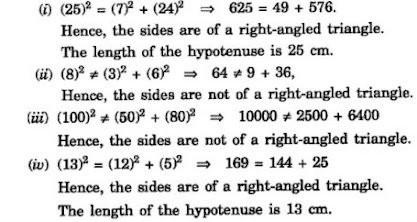2. PQR is a triangle right angled at P and M is a point on QR such that PM ⊥ QR. Show that PM2 = QM × MR.

3. In Figure, ABD is a triangle right angled at A and AC ⊥ BD. Show that
(i) AB2 = BC × BD
(ii) AC2 = BC × DC
(iii) AD2 = BD × CD

4. ABC is an isosceles triangle right angled at C. Prove that AB2 = 2AC2 .

5. ABC is an isosceles triangle with AC = BC. If AB2 = 2AC2, prove that ABC is a right triangle.

6. ABC is an equilateral triangle of side 2a. Find each of its altitudes.

7. Prove that the sum of the squares of the sides of rhombus is equal to the sum of the squares of its diagonals.

8. In Fig. 6.54, O is a point in the interior of a triangle.

9. A ladder 10 m long reaches a window 8 m above the ground. Find the distance of the foot of the ladder from base of the wall.

10. A guy wire attached to a vertical pole of height 18 m is 24 m long and has a stake attached to the other end. How far from the base of the pole should the stake be driven so that the wire will be taut?

11. An aeroplane leaves an airport and flies due north at a speed of 1,000 km per hour. At the same time, another aeroplane leaves the same airport and flies due west at a speed of 1,200 km per hour. How far apart will be the two planes after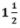hours?

Solution

12. Two poles of heights 6 m and 11 m stand on a plane ground. If the distance between the feet of the poles is 12 m, find the distance between their tops.

13. D and E are points on the sides CA and CB respectively of a triangle ABC right angled at C. Prove that AE2 + BD2 = AB2 + DE2.

15. In an equilateral triangle ABC, D is a point on side BC such that BD = 1/3BC. Prove that 9AD2 = 7AB2.

16. In an equilateral triangle, prove that three times the square of one side is equal to four times the square of one of its altitudes.

17. Tick the correct answer and justify: In ΔABC, AB = 6√3 cm, AC = 12 cm and BC = 6 cm.
The angle B is:
(A) 120°
(B) 60°
(C) 90°
(D) 45°

#### Exercise 6.6

1. In Figure, PS is the bisector of ∠ QPR of ∆ PQR. Prove that QS/PQ = SR/PR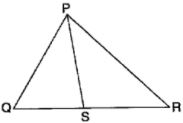2. In Fig. 6.57, D is a point on hypotenuse AC of ∆ABC, such that BD ⊥AC, DM ⊥ BC and DN ⊥ AB. Prove that: (i) DM2 = DN . MC (ii) DN2 = DM . AN.

Solution

3. In Figure, ABC is a triangle in which ∠ABC > 90° and AD ⊥ CB produced. Prove that
AC2= AB2+ BC2+ 2 BC.BD.

4. In Figure, ABC is a triangle in which ∠ ABC < 90° and AD ⊥ BC. Prove that
AC2= AB2+ BC2 – 2 BC.BD.

5. In Figure, AD is a median of a triangle ABC and AM ⊥ BC. Prove that :
(i) AC2 = AD2 + BC.DM + 2 (BC/2) 2
(ii) AB2 = AD2 – BC.DM + 2 (BC/2) 2
(iii) AC2 + AB2 = 2 AD2 + ½ BC2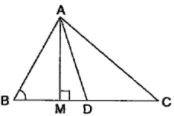6. Prove that the sum of the squares of the diagonals of parallelogram is equal to the sum of the squares of its sides.

7. In Figure, two chords AB and CD intersect each other at the point P. Prove that :
(i) ∆APC ~ ∆ DPB
(ii) AP . PB = CP . DP

8. In Fig. 6.62, two chords AB and CD of a circle intersect each other at the point P (when produced) outside the circle. Prove that:
(i) ∆ PAC ~ ∆ PDB
(ii) PA . PB = PC . PD.

9. In Figure, D is a point on side BC of ∆ ABC such that BD/CD = AB/AC. Prove that AD is the bisector of ∠ BAC.